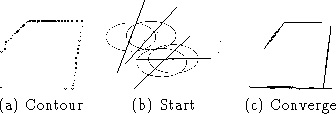Next: Symmetry Detection Up: Vision Processing Previous: Color Feature Detection

## Contour Computation

We are particularly interested in the contour of the pool table since this identifies its shape and periphery where the pockets lie. We quickly compute the centroid of the pool table and compute the most radially distant points at each angle from the center as in Figure(a).

Since we know that the pool table top is a quadrilateral object we model its contour as a set of 4 lines. This is done by fitting the distribution of contour pixels using an EM algorithm where each sampleis a (u,v) vector. In this vector, u is the horizontal coordinate and v is the vertical coordinate of the contour pixel. A mixture model consisting of four Gaussians is updating using EM as shown in Figure(b) and (c). The model converges so that the thin Gaussians form lines that approximate the sides of the table. The dominant eigenvector of each Gaussian is along its main axis and is used to compute a linear model as shown.Figure: Pool Table Contour Lines via EM

Thus, we have four line equations describing the boundary of the pool table in each image frame. It is possible to compute intersections to find pool table corners and to determine the lengths of the sides. In addition, we can accurately specify the interior and periphery of the pool table.

Tony Jebara
Wed Feb 18 18:52:15 EST 1998